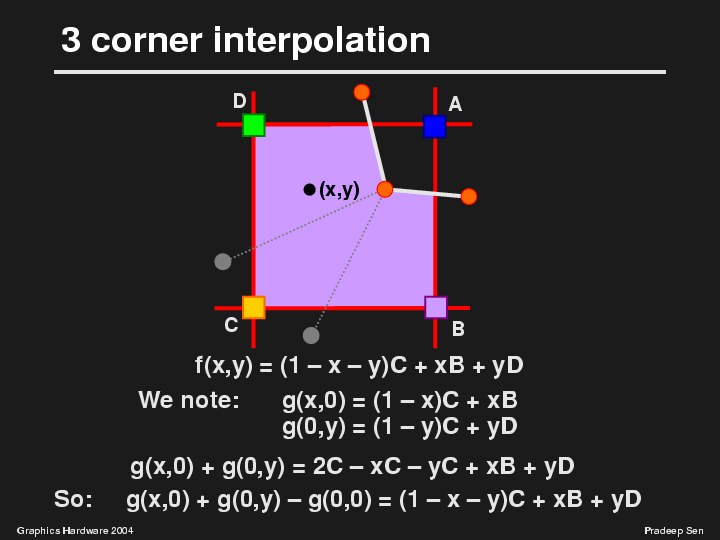Silhouette Maps for Improved Texture Magnification

 Slide 44 of 61 [index] [first] [prev] [next]The interpolation function we want to perform is shown here as f(x,y), and is simply barycentric interpolation. It is also the result you would get if you fit a plane through the three corner points to try to determine the value of the point to shade. Please note that we have to be careful about clamping the result when it extends beyond the B,C,D triangle and we discuss that in the paper. So how do we implement this function? We note that we have two linear interpolators available to us through the bilinear interpolation function g(x,y). The first is the linear interpolation between C and B, which we get if we simply plug in the x-coordinate into g(x,y) and zero out the y input. The second is the linear interpolation between C and D that can be computed if we keep the y-coordinate of the bilinear interpolation function and zero out the x input. Now we note that if we add these two functions, we get an expression that is very similar to what we want, except that it has an extra "C" term. Thus, we can subtract out the "C" term (in this case by subtracting out g(0,0) since the origin of the cell is defined to be the C corner) to get the expression we want, f(x,y). This means that it is possible to express the 3-corner case in terms of a combination of 3 bilinear interpolations.

As presented at SIGGRAPH/EUROGRAPHICS Graphics Hardware 2004
by Pradeep Sen on August 30, 2004